In general, writing a comment means writing some text that defines your feeling, opinion, or whatever you say about any particular thing. In the same way, a comment in a Python program can be used to explain a code, a statement, or the whole program.

### What is a comment in Python?

So we can say that a comment is basically a human-readable explanation or annotation in the Python program. It is possible to explain:

• any particular statement
• any block of code
• any piece of code
• sometimes the whole program
• or whatever the programmer needs to explanation the thing in the program.

Note: Comment can also be used to comment out any code or block of code to skip its execution by the compiler. Therefore, a comment can also be used for practice or debugging.

Note: Generally, comments are used to provide in-program descriptions of the code.

### Why do we use comments in Python?

Most of the time, comments in Python are used to make source code easier for other programmers to understand, or even for the creator of the program to understand, while reading the code now or later.

Another use of a comment in a Python program is if you find that a block of code in your program is not executing or performing its work in the correct way. Then, instead of deleting that block of code, you can comment it out and create another block of code. Maybe the error was found while making a new block of code, so just remove the "comment" from the old block of code and change it.

Commenting also helps in reviewing the Python code later. So, this article tells you all about the use of comments in Python programming, along with an example program.

## Types of Comment in Python

The Python programming language provides two types of comments to use in the source code of the program, namely:

## Single-line comment in Python

A single-line comment in Python is a comment that is written after the hash symbol (#). That is, all characters after the hash (#) sign are referred to as comments (single-line comments), up to the physical line end. For example:

```# I'm a single-line comment in Python

print("Hey!\nHow are you?")

# I'm also a single-line comment in Python```

This program produces exactly the same output as shown in the snapshot given below: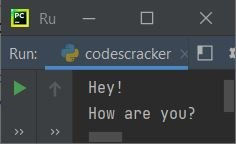As you can see from the above program and its output, only the statement:

`print("Hey!\nHow are you?")`

gets executed. Before saying anything further, let's create another program:

```# I'm a single-line comment in Python

# print("Hey!\nHow are you?")

print("Comment Tutorial in Python Programming")

# I'm also a single-line comment in Python```

This time, the program produces the following output: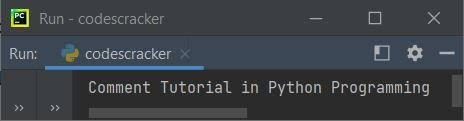You saw how the statement:

`print("Hey!\nHow are you?")`

gets skipped for execution by the compiler only because of the # (hash symbol) added before the statement. These are just some demo programs that show how to comment something in Python source code. Let's create another program that uses comments to describe some steps of the program:

```print("Enter a Number: ")

# input() converts the entered value into a string by default.
a = input()

print("Enter another Number: ")
b = input()

# int() converts a string to an integer.
a = int(a)
b = int(b)

sum = a+b

print("\nSum =", sum)```

The snapshot given below shows the sample run of the above program, with user inputs 12 and 34 as the first and second numbers: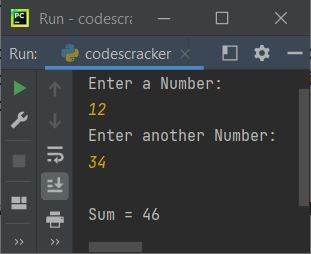See how comments can be useful sometimes to provide in-program descriptions of all the codes. If you remove all the # (hash symbol) to uncomment the thing from the above program, That is, if the above program gets replaced with:

```print("Enter a Number: ")

input converts the entered value into a string by default.
a = input()

print("Enter another Number: ")
b = input()

int() converts a string to an integer.
a = int(a)
b = int(b)

sum = a+b

print("\nSum =", sum)```

Then the un-comment part also gets executed by the compiler. And you'll see some syntax error messages in this case, as shown in the snapshot given below:So it is proven that comments are ignored by the compiler. Now let's talk about multi-line comments, and then I will summarize everything.

## Multi-line comment in Python

A multi-line comment is one that is written or expanded on multiple lines rather than just one.In Python, for multi-line comments, we use """ (triple quotes) or ''' (triple apostrophe). For example:

```''' This is a
multi-line comment
in Python
'''

print("Hey\nHow're You?")

""" This is also a
multi-line comment
in Python
"""```

If you run the above Python code, then you will see the following output on your output screen: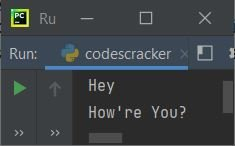The above program can also be written as:

```''' This is a
multi-line comment
in Python '''

print("Hey\nHow're You?")

""" This is also a
multi-line comment
in Python
"""```

That is, how you write text inside the triple quote or apostrophe is entirely up to you. Let's create another program that uses multi-line comments to provide an in-program description of the source code:

```print("Enter a Number: ")

''' By default, input() converts the entered value into a string.
So when the user enters 12 as input,
it gets treated as a string.
That is, a = "12."
'''
a = input()

print("Enter another Number: ")
b = input()

''' Since a = "12,"
say, a and b, gives "12" + "34."
That is, "1234."
If the user enters 34 as the second number,
'''
print("\nSum = ", a+b)

''' Strings are converted to integers
using the int() function.
So int(a) or int("12") returns 12.
Similarly, int(b) or int("34") gives 34.
'''
a = int(a)
b = int(b)

sum = a+b
print("\nSum =", sum)

""" Since the first parameter of print() above
is of the string type,
as a result,
the second string must be of string type
in order to be concatenated using +,
as shown below.
The str() method transforms any type into a string.
"""
print("\nSum = " + str(sum))```

Here is its sample run with user input of 12 and 34 as two numbers: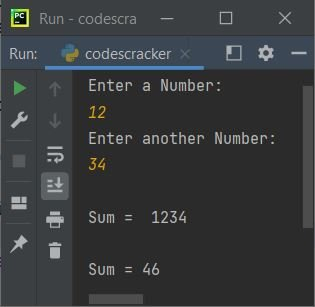See how easily multi-line comments can be used to provide in-program descriptions of the code?
Because I used the multi-line comment to describe each and every piece of code used in the preceding program, there is no need to expand on the description. If any user, including yourself, sees the program now or later, he or she, as well as you, will easily understand all of the code using the provided comment.

### Using a single-line comment as a multi-line comment in Python

To use a single-line comment as a multi-line comment in Python, we need to add the # (hash) sign at the start of each comment line. For example:

```# This is a single-line comment in Python
# The single-line comment is used here as a multi-line comment

print("Hey!")```

The disadvantage of using a single-line comment as a multi-line comment is that the hash (#) sign must be written before each line of the comment.

### In Python, using a multi-line comment as a single-line comment

The example given below shows how to use a multi-line comment as a single-line comment in Python:

```''' As a single-line comment, this is a multi-line comment. '''

print("Hey!")```

If comments are written just after the function, class, or method using multi-line comments (''' or """"). Then the comment can be accessed using the __doc__ attribute, as shown in the program and its sample output given below:

```def codescracker():
""" Hey,
This is a multi-line comment
written in the codescracker() function.
"""

print(codescracker.__doc__)```

Here is the output produced by the above program: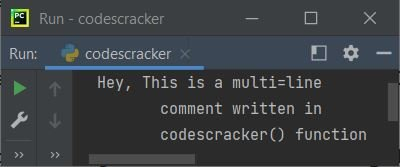Note: The way you write the code, including spaces, gets treated as being inside the PRE tag of HTML. That is, everything, including spaces and newlines, and of course the text itself, gets accessed using the __doc__ attribute.

Let's modify the above program with the one given below:

```def codescracker():
""" Hey,
This is a multi-line comment
written in the codescracker() function.
"""

print(codescracker.__doc__)```

produces the following output:Python Online Test

« Previous Tutorial Next Tutorial »